# ISEE Upper Level Math : How to find the angle of clock hands

## Example Questions

### Example Question #1 : How To Find The Angle Of Clock Hands

At which of the following times on a clock is the smaller angle created by the minute hand and hour hand NOT less than?

11:00

2:00

4:30

1:30

1:30

Explanation:

In this problem, you are working with the shape of a clock. A clock is in the shape of a circle, which has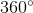total. You are looking for the time for which the smaller angle created by the minute and hour hands is NOT less than- in other words, the time must create an angle greater than.

First, it's helpful to recognize at what times a right angle () exists. This would be 3:00 and 9:00. It's also useful to recognize that times such as 3:30 and 9:30 will be slightly under- the hour hand moves just past the 3 and 9 on a clock at these times to create this effect. You can draw a clock out to show these angles.

The three times that do create an angle less than, and thus are incorrect, are 2:00, 4:30, and 11:00.

2:00 has the minute hand straight up on the 12 and the hour hand straight at the 2, making it less than theangle created by 3:00.

4:30 has the hour hand in between the 4 and 5 and the minute hand on the 6, making it less than theangle created by 3:30.

11:00 has the minute hand straight up on the 12 and the hour hand straight at the 11, making it less than theangle created by 9:00.

1:30 is the angle that is NOT less than. The hour hand is in between the 1 and 2, and the minute hand is straight down on the 6. This angle is larger than a perfect right angle of. Therefore, 1:30 is the correct answer.

### Example Question #2 : How To Find The Angle Of Clock Hands

How many degrees are in an angle formed by the hour and minute hand when it is 5:15?Explanation:

When it is 5:15, the hour hand is pointed to 5 and the minute hand is pointed to 3.

Therefore, the angle created will span between 3 and 5, which spans "2 hours" worth of time on the clock. Given that there are a total of 12 hours of time on the clock, this means that the angle formed will be equal to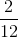of the total degrees in the clock.

Since the clock is a circle, it contains 360 degrees.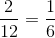of 360 is 60; therefore, 60 is the correct answer.

### Example Question #3 : How To Find The Angle Of Clock Hands

How many degrees are are in the angle formed by the minute and hour hand when it is 7:05?Explanation:

When it is 7:05, the hour hand is pointed to 7 and the minute hand is pointed to 1.

Therefore, the angle created will span between 1 and 7, which spans "6 hours" worth of time on the clock. Given that there are a total of 12 hours of time on the clock, this means that the angle formed will be equal to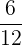of the total degrees in the clock.

Since the clock is a circle, it contains 360 degrees.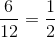of 360 is 180; therefore, 180 is the correct answer.

### Example Question #4 : How To Find The Angle Of Clock Hands

The hour hand and the minute hand of a clock form a 30-degree angle. If the minute hand reads 55 minutes, what number may the hour hand be pointed to?

11 or 12

10 or 11

10 or 12

11 or 1

10 or 12

Explanation:

When the minute hand is pointed to 55 minutes (or the 11th hour), the hour hand must be pointed to either 10 or 12.

This is because the angle created will span between 10 and 11 or 11 and 12, which spans "1 hour" worth of time on the clock. Given that there are a total of 12 hours of time on the clock, this means that the angle formed will be equal to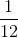of the total degrees in the clock.

Since the clock is a circle, it contains 360 degrees.of 360 is 30; therefore, the answer choice 10 or 12 proves to be correct.

### Example Question #5 : How To Find The Angle Of Clock Hands

The hour hand and the minute hand of a clock form a 60-degree angle. If the minute hand reads 45 minutes, what number may the hour hand be pointed to?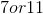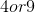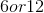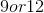Explanation:

When the hour hand is pointed to 45 minutes (or the 9th hour), the hour hand must be pointed to either 7 or 11.

This is because the angle created will span between 9 and 11 or 9 and 7, which spans "2 hours" worth of time on the clock. Given that there are a total of 12 hours of time on the clock, this means that the angle formed will be equal toof the total degrees in the clock.

Since the clock is a circle, it contains 360 degrees.of 360 is 60; therefore, the answer choice 7 or 11 proves to be correct.